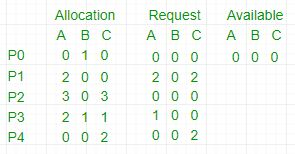Open in App
Not now

# Deadlock Detection Algorithm in Operating System

• Difficulty Level : Medium
• Last Updated : 17 Feb, 2023

The algorithm employs several times varying data structures:

• Available –
A vector of length m indicates the number of available resources of each type.
• Allocation –
An n*m matrix defines the number of resources of each type currently allocated to a process. The column represents resource and rows represent a process.
• Request –
An n*m matrix indicates the current request of each process. If request[i][j] equals k then process Pi is requesting k more instances of resource type Rj.

This algorithm has already been discussed here

Now, the Bankers algorithm includes a Safety Algorithm / Deadlock Detection Algorithm
The algorithm for finding out whether a system is in a safe state can be described as follows:

Steps of Algorithm:

1. Let Work and Finish be vectors of length m and n respectively. Initialize Work= Available. For i=0, 1, …., n-1, if Requesti = 0, then Finish[i] = true; otherwise, Finish[i]= false.
2. Find an index i such that both
a) Finish[i] == false
b) Requesti <= Work
If no such i exists go to step 4.
3. Work= Work+ Allocationi
Finish[i]= true
Go to Step 2.
4. If Finish[i]== false for some i, 0<=i<n, then the system is in a deadlocked state. Moreover, if Finish[i]==false the process Pi is deadlocked.

For example,1. In this, Work = [0, 0, 0] &
Finish = [false, false, false, false, false]

2. i=0 is selected as both Finish = false and [0, 0, 0]<=[0, 0, 0].

3. Work =[0, 0, 0]+[0, 1, 0] =>[0, 1, 0] &
Finish = [true, false, false, false, false].

4. i=2 is selected as both Finish = false and [0, 0, 0]<=[0, 1, 0].
5. Work =[0, 1, 0]+[3, 0, 3] =>[3, 1, 3] &
Finish = [true, false, true, false, false].

6. i=1 is selected as both Finish = false and [2, 0, 2]<=[3, 1, 3].
7. Work =[3, 1, 3]+[2, 0, 0] =>[5, 1, 3] &
Finish = [true, true, true, false, false].

8. i=3 is selected as both Finish = false and [1, 0, 0]<=[5, 1, 3].
9. Work =[5, 1, 3]+[2, 1, 1] =>[7, 2, 4] &
Finish = [true, true, true, true, false].

10. i=4 is selected as both Finish = false and [0, 0, 2]<=[7, 2, 4].

11. Work =[7, 2, 4]+[0, 0, 2] =>[7, 2, 6] &
Finish = [true, true, true, true, true].

12. Since Finish is a vector of all true it means there is no deadlock in this example.

### There are several algorithms for detecting deadlocks in an operating system, including:

1. Wait-For Graph: A graphical representation of the system’s processes and resources. A directed edge is created from a process to a resource if the process is waiting for that resource. A cycle in the graph indicates a deadlock.
2. Banker’s Algorithm: A resource allocation algorithm that ensures that the system is always in a safe state, where deadlocks cannot occur.
3. Resource Allocation Graph: A graphical representation of processes and resources, where a directed edge from a process to a resource means that the process is currently holding that resource. Deadlocks can be detected by looking for cycles in the graph.
4. Detection by System Modeling: A mathematical model of the system is created, and deadlocks can be detected by finding a state in the model where no process can continue to make progress.
5. Timestamping: Each process is assigned a timestamp, and the system checks to see if any process is waiting for a resource that is held by a process with a lower timestamp.

These algorithms are used in different operating systems and systems with different resource allocation and synchronization requirements. The choice of algorithm depends on the specific requirements of the system and the trade-offs between performance, complexity, and accuracy.

If a system does not employ either a deadlock prevention or deadlock avoidance algorithm then a deadlock situation may occur. In this case-

• Apply an algorithm to examine the system’s state to determine whether deadlock has occurred.
• Apply an algorithm to recover from the deadlock. For more refer- Deadlock Recovery

1. Improved System Stability: Deadlocks are a major concern in operating systems, and detecting and resolving deadlocks can help to improve the stability of the system.
2. Better Resource Utilization: By detecting deadlocks and freeing resources, the operating system can ensure that resources are efficiently utilized and that the system remains responsive to user requests.
3. Easy Implementation: Some deadlock detection algorithms, such as the Wait-For Graph, are relatively simple to implement and can be used in a wide range of operating systems and systems with different resource allocation and synchronization requirements.